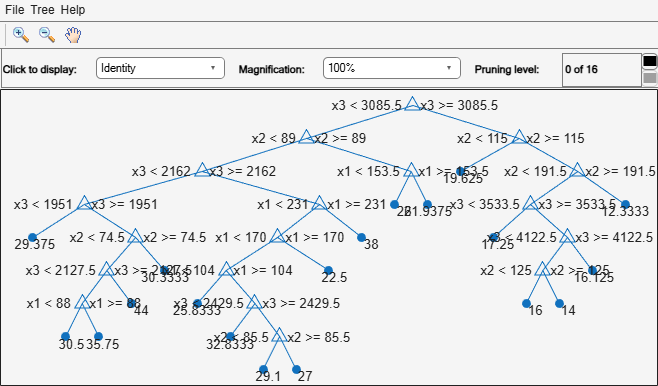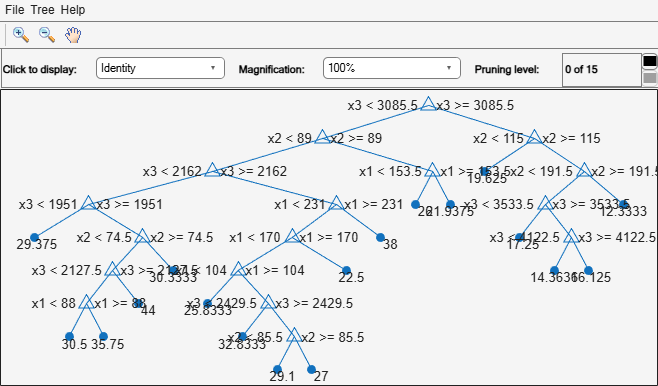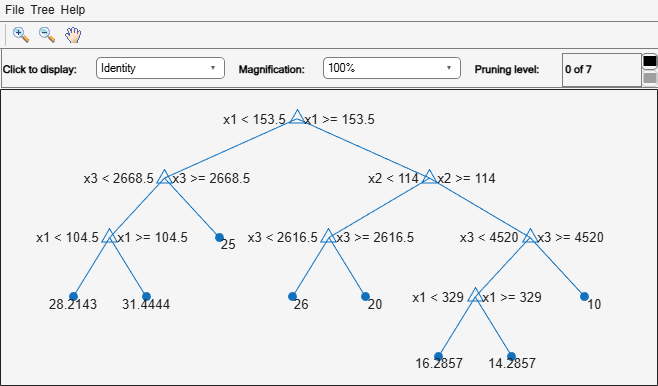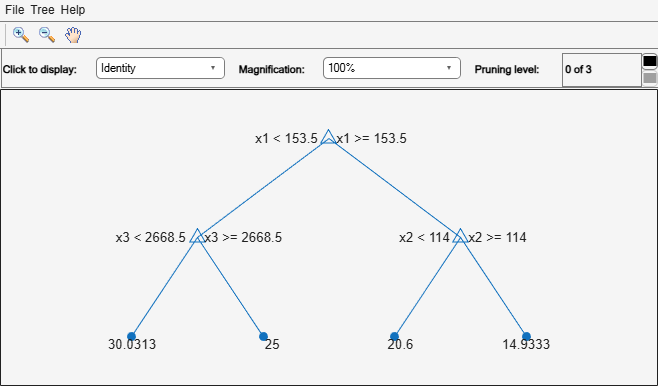# loss

Regression error

## Syntax

``L = loss(tree,Tbl,ResponseVarName)``
``L = loss(tree,Tbl,Y)``
``L = loss(tree,X,Y)``
``L = loss(___,Name=Value)``
``````[L,se,NLeaf,bestLevel] = loss(___)``````

## Description

example

````L = loss(tree,Tbl,ResponseVarName)` returns the mean squared error between the predictions of `tree` to the data in `Tbl`, compared to the true responses `Tbl.ResponseVarName`.```
````L = loss(tree,Tbl,Y)` returns the mean squared error between the predictions of `tree` to the data in `Tbl`, compared to the true responses `Y`.```
````L = loss(tree,X,Y)` returns the mean squared error between the predictions of `tree` to the data in `X`, compared to the true responses `Y`.```
````L = loss(___,Name=Value)` computes the error in prediction with additional options specified by one or more name-value arguments, using any of the previous syntaxes.```

example

``````[L,se,NLeaf,bestLevel] = loss(___)``` also returns the standard error of the loss (`se`), the number of leaves (terminal nodes) in the tree (`NLeaf`), and the optimal pruning level for `tree` (`bestLevel`).```

## Examples

collapse all

Load the `carsmall` data set. Consider `Displacement`, `Horsepower`, and `Weight` as predictors of the response `MPG`.

```load carsmall X = [Displacement Horsepower Weight];```

Grow a regression tree using all observations.

`tree = fitrtree(X,MPG);`

Estimate the in-sample MSE.

`L = loss(tree,X,MPG)`
```L = 4.8952 ```

Load the `carsmall` data set. Consider `Displacement`, `Horsepower`, and `Weight` as predictors of the response `MPG`.

```load carsmall X = [Displacement Horsepower Weight];```

Grow a regression tree using all observations.

`Mdl = fitrtree(X,MPG);`

View the regression tree.

`view(Mdl,Mode="graph");`Find the best pruning level that yields the optimal in-sample loss.

```[L,se,NLeaf,bestLevel] = loss(Mdl,X,MPG,Subtrees="all"); bestLevel```
```bestLevel = 1 ```

The best pruning level is level 1.

Prune the tree to level 1.

```pruneMdl = prune(Mdl,Level=bestLevel); view(pruneMdl,Mode="graph");```Unpruned decision trees tend to overfit. One way to balance model complexity and out-of-sample performance is to prune a tree (or restrict its growth) so that in-sample and out-of-sample performance are satisfactory.

Load the `carsmall` data set. Consider `Displacement`, `Horsepower`, and `Weight` as predictors of the response `MPG`.

```load carsmall X = [Displacement Horsepower Weight]; Y = MPG;```

Partition the data into training (50%) and validation (50%) sets.

```n = size(X,1); rng(1) % For reproducibility idxTrn = false(n,1); idxTrn(randsample(n,round(0.5*n))) = true; % Training set logical indices idxVal = idxTrn == false; % Validation set logical indices```

Grow a regression tree using the training set.

`Mdl = fitrtree(X(idxTrn,:),Y(idxTrn));`

View the regression tree.

`view(Mdl,Mode="graph");`The regression tree has seven pruning levels. Level 0 is the full, unpruned tree (as displayed). Level 7 is just the root node (i.e., no splits).

Examine the training sample MSE for each subtree (or pruning level) excluding the highest level.

```m = max(Mdl.PruneList) - 1; trnLoss = resubLoss(Mdl,SubTrees=0:m)```
```trnLoss = 7×1 5.9789 6.2768 6.8316 7.5209 8.3951 10.7452 14.8445 ```
• The MSE for the full, unpruned tree is about 6 units.

• The MSE for the tree pruned to level 1 is about 6.3 units.

• The MSE for the tree pruned to level 6 (i.e., a stump) is about 14.8 units.

Examine the validation sample MSE at each level excluding the highest level.

`valLoss = loss(Mdl,X(idxVal,:),Y(idxVal),Subtrees=0:m)`
```valLoss = 7×1 32.1205 31.5035 32.0541 30.8183 26.3535 30.0137 38.4695 ```
• The MSE for the full, unpruned tree (level 0) is about 32.1 units.

• The MSE for the tree pruned to level 4 is about 26.4 units.

• The MSE for the tree pruned to level 5 is about 30.0 units.

• The MSE for the tree pruned to level 6 (i.e., a stump) is about 38.5 units.

To balance model complexity and out-of-sample performance, consider pruning `Mdl` to level 4.

```pruneMdl = prune(Mdl,Level=4); view(pruneMdl,Mode="graph")```## Input Arguments

collapse all

Trained regression tree, specified as a `RegressionTree` object created by the `fitrtree` function or a `CompactRegressionTree` object created by the `compact` function.

Sample data, specified as a table. Each row of `Tbl` corresponds to one observation, and each column corresponds to one predictor variable. `Tbl` must contain all of the predictors used to train `tree`. Optionally, `Tbl` can contain additional columns for the response variable and observation weights. Multicolumn variables and cell arrays other than cell arrays of character vectors are not allowed.

If `Tbl` contains the response variable used to train `tree`, then you do not need to specify `ResponseVarName` or `Y`.

If you trained `tree` using sample data contained in a table, then the input data for this method must also be in a table.

Data Types: `table`

Predictor values, specified as a numeric matrix. Each column of `X` represents one variable, and each row represents one observation.

`X` must have the same number of columns as the data used to train `tree`. `X` must have the same number of rows as the number of elements in `Y`.

Data Types: `single` | `double`

Response variable name, specified as the name of a variable in `Tbl`. If `Tbl` contains the response variable used to train `tree`, then you do not need to specify `ResponseVarName`.

If you specify `ResponseVarName`, then you must do so as a character vector or string scalar. For example, if the response variable is stored as `Tbl.Response`, then specify it as `"Response"`. Otherwise, the software treats all columns of `Tbl`, including `Tbl.ResponseVarName`, as predictors.

Data Types: `char` | `string`

Response data, specified as a numeric column vector with the same number of rows as `X`. Each entry in `Y` is the response to the data in the corresponding row of `X`.

Data Types: `single` | `double`

### Name-Value Arguments

Specify optional pairs of arguments as `Name1=Value1,...,NameN=ValueN`, where `Name` is the argument name and `Value` is the corresponding value. Name-value arguments must appear after other arguments, but the order of the pairs does not matter.

Example: `L = loss(tree,X,Y,Subtrees="all")` prunes all subtrees.

Before R2021a, use commas to separate each name and value, and enclose `Name` in quotes.

Loss function, specified as a function handle for loss, or `"mse"` representing mean-squared error. If you pass a function handle `fun`, `loss` calls `fun` as:

`fun(Y,Yfit,W)`
• `Y` is the vector of observed responses.

• `Yfit` is the vector of predicted responses.

• `W` is the observation weights. If you pass `W`, the elements are normalized to sum to `1`.

All the vectors have the same number of rows as `Y`.

Example: `LossFun="mse"`

Data Types: `function_handle` | `char` | `string`

Pruning level, specified as a vector of nonnegative integers in ascending order or `"all"`.

If you specify a vector, then all elements must be at least `0` and at most `max(tree.PruneList)`. `0` indicates the full, unpruned tree and `max(tree.PruneList)` indicates the completely pruned tree (in other words, just the root node).

If you specify `"all"`, then `loss` operates on all subtrees (in other words, the entire pruning sequence). This specification is equivalent to using `0:max(tree.PruneList)`.

`loss` prunes `tree` to each level indicated in `Subtrees`, and then estimates the corresponding output arguments. The size of `Subtrees` determines the size of some output arguments.

To invoke `Subtrees`, the properties `PruneList` and `PruneAlpha` of `tree` must be nonempty. In other words, grow `tree` by setting `Prune="on"`, or by pruning `tree` using `prune`.

Example: `Subtrees="all"`

Data Types: `single` | `double` | `char` | `string`

Tree size, specified as one of the following:

• `"se"` — The `loss` function returns `bestLevel` that corresponds to the smallest tree whose mean squared error (MSE) is within one standard error of the minimum MSE.

• `"min"` — The `loss` function returns `bestLevel` that corresponds to the minimal MSE tree.

Example: `TreeSize="min"`

Observation weights, specified as a numeric vector or the name of a variable in `Tbl`. The software weights the observations in each row in `X` or `Tbl` with the corresponding value in `Weights`. The length of `Weights` must equal the number of rows in `X` or `Tbl`.

If you specify the input data as a table `Tbl`, then you can specify `Weights` as the name of a variable in `Tbl` that contains a numeric vector. In this case, you must specify `Weights` as a string scalar or character vector. For example, if weights vector `W` is stored as `Tbl.W`, then specify `Weights` as `"W"`. Otherwise, the software treats all columns of `Tbl`, including `W`, as predictors when training the model.

Data Types: `single` | `double` | `char` | `string`

## Output Arguments

collapse all

Classification error, returned as a numeric vector of the length of `Subtrees`. The error for each tree is the mean squared error, weighted with `Weights`. If you include `LossFun`, then `L` reflects the loss calculated with `LossFun`.

Standard error of loss, returned as a vector of the length of `Subtrees`.

Number of leaves in the pruned subtrees, returned as a numeric vector of the length of `Subtrees`. Leaf nodes are terminal nodes, which give responses, not splits.

Best pruning level as defined in the `TreeSize` name-value argument, returned as a numeric scalar whose value depends on `TreeSize`:

• When `TreeSize` is `"se"`, the `loss` function returns the highest pruning level with loss within one standard deviation of the minimum (`L`+`se`, where `L` and `se` relate to the smallest value in `Subtrees`).

• When `TreeSize` is `"min"`, the `loss` function returns the element of `Subtrees` with smallest loss, usually the smallest element of `Subtrees`.

collapse all

### Mean Squared Error

The mean squared error m of the predictions f(Xn) with weight vector w is

`$m=\frac{\sum {w}_{n}{\left(f\left({X}_{n}\right)-{Y}_{n}\right)}^{2}}{\sum {w}_{n}}.$`

## Version History

Introduced in R2011a